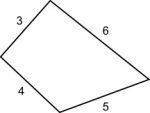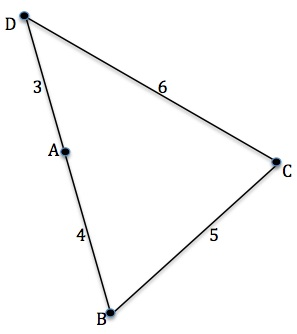Four rods are hinged at their ends to form a convex quadrilateral with sides of length 3, 4, 5 and 6 (in that order). Investigate the different shapes that the quadrilateral can take if the polygon is always convex.

How do the angles change as the bendy quad changes shape?

Can any of the angles reduce to zero degrees?

Can any of the angles increase to 180 degrees?Calculate the size of angles B,  C and D when the rods form a triangle as shown.

If the polygon remains convex, can angle C get any larger than shown in this diagram ? Can angle B get any smaller? Can angle D get any smaller? So what values can the angles take?

Can the quadrilateral be cyclic? If so what are its angles?